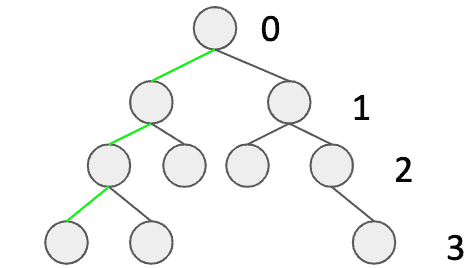## 1. Overview

Trees are one of the most important data structures in computer science. We’re usually interested in a balanced tree, because of its valuable properties. Their structure allows performing operations like queries, insertions, deletions in logarithmic time.

In this tutorial, we’re going to learn how to determine if a binary tree is balanced.

## 2. Definitions

First, let’s introduce a few definitions in order to make sure we’re on the same page:

• A binary tree – a kind of a tree where every node has zero, one or two children
• A height of a tree – a maximum distance from a root to a leaf (same as the depth of the deepest leaf)
• A balanced tree – a kind of a tree where for every subtree the maximum distance from the root to any leaf is at most bigger by one than the minimum distance from the root to any leaf

We can find an example of a balanced binary tree below. Three green edges are a simple visualization of how to determine the height, while the numbers indicate the level.## 3. Domain Objects

``````public class Tree {
private int value;
private Tree left;
private Tree right;

public Tree(int value, Tree left, Tree right) {
this.value = value;
this.left = left;
this.right = right;
}
}
``````

For the sake of simplicity, let’s say each node has an integer value. Note, that if left and right trees are null, then it means our node is a leaf.

Before we introduce our primary method let’s see what it should return:

``````private class Result {
private boolean isBalanced;
private int height;

private Result(boolean isBalanced, int height) {
this.isBalanced = isBalanced;
this.height = height;
}
}``````

Thus for every single call, we’ll have information about height and balance.

## 4. Algorithm

Having a definition of a balanced tree, we can come up with an algorithm. What we need to do is to check the desired property for every node. It can be achieved easily with recursive depth-first search traversal.

Now, our recursive method will be invoked for every node. Additionally, it will keep track of the current depth. Each call will return information about height and balance.

Now, let’s take a look at our depth-first method:

``````private Result isBalancedRecursive(Tree tree, int depth) {
if (tree == null) {
return new Result(true, -1);
}

Result leftSubtreeResult = isBalancedRecursive(tree.left(), depth + 1);
Result rightSubtreeResult = isBalancedRecursive(tree.right(), depth + 1);

boolean isBalanced = Math.abs(leftSubtreeResult.height - rightSubtreeResult.height) <= 1;
boolean subtreesAreBalanced = leftSubtreeResult.isBalanced && rightSubtreeResult.isBalanced;
int height = Math.max(leftSubtreeResult.height, rightSubtreeResult.height) + 1;

return new Result(isBalanced && subtreesAreBalanced, height);
}``````

First, we need to consider the case if our node is null: we’ll return true (which means the tree is balanced) and -1 as a height.

Then, we make two recursive calls for the left and the right subtree, keeping the depth up to date.

At this point, we have calculations performed for children of a current node. Now, we have all the required data to check balance:

• the isBalanced variable checks the height for children, and
• substreesAreBalanced indicates if the subtrees are both balanced as well

Finally, we can return information about balance and height. It might be also a good idea to simplify the first recursive call with a facade method:

``````public boolean isBalanced(Tree tree) {
return isBalancedRecursive(tree, -1).isBalanced;
}``````

## 5. Summary

In this article, we’ve discussed how to determine if a binary tree is balanced. We’ve explained a depth-first search approach.

As usual, the source code with tests is available over on GitHub.

### Get started with Spring and Spring Boot, through the Learn Spring course:

>> CHECK OUT THE COURSE